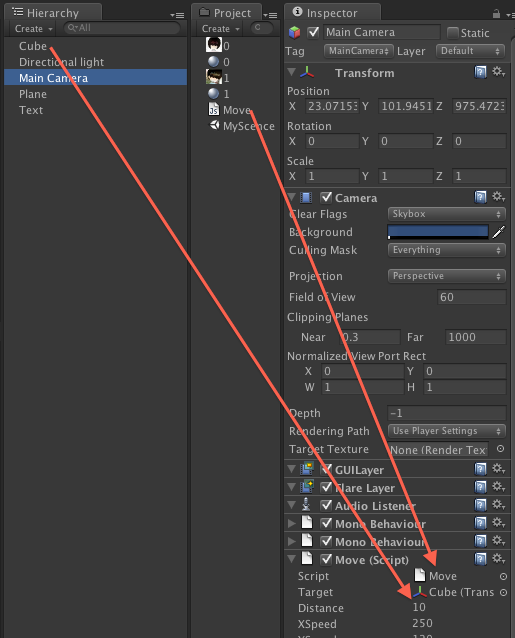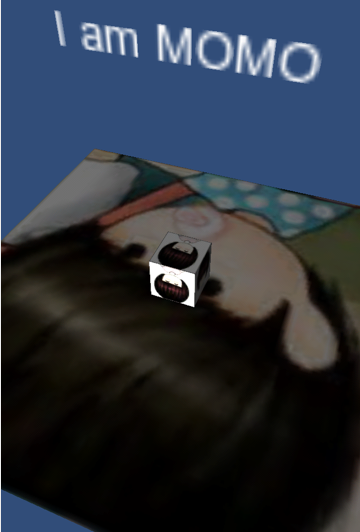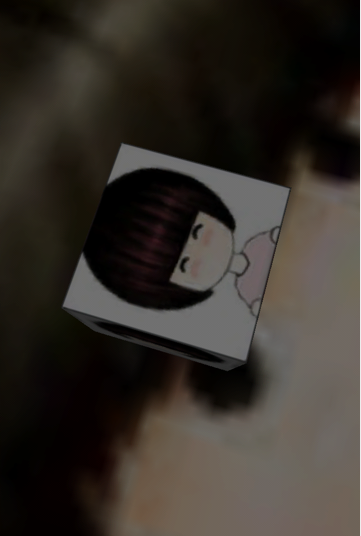## Unity3D 游戏引擎之IOS触摸屏手势控制镜头旋转与缩放（八）

为什么80%的码农都做不了架构师？->>>Unity3D 游戏引擎之IOS触摸屏手势控制镜头旋转与缩放function Start () ： 游戏启动以后只调用一次，可用于脚本的初始化操作，

function Update ()：Start()方法调用结束以后每一帧都会调用，可以在这里更新游戏逻辑。

function LateUpdate (): Start()方法调用结束以后每一帧都会调用，但是它是在 Update()调用完后调用。

Move.js完整代码

```//用于绑定参照物对象
var target : Transform;
//缩放系数
var distance = 10.0;
//左右滑动移动速度
var xSpeed = 250.0;
var ySpeed = 120.0;
//缩放限制系数
var yMinLimit = -20;
var yMaxLimit = 80;
//摄像头的位置
var x = 0.0;
var y = 0.0;
//记录上一次手机触摸位置判断用户是在左放大还是缩小手势
private var oldPosition1 : Vector2;
private var oldPosition2 : Vector2;

//初始化游戏信息设置
function Start () {
var angles = transform.eulerAngles;
x = angles.y;
y = angles.x;

// Make the rigid body not change rotation
if (rigidbody)
rigidbody.freezeRotation = true;
}

function Update ()
{
//判断触摸数量为单点触摸
if(Input.touchCount == 1)
{
//触摸类型为移动触摸
if(Input.GetTouch(0).phase==TouchPhase.Moved)
{
//根据触摸点计算X与Y位置
x += Input.GetAxis("Mouse X") * xSpeed * 0.02;
y -= Input.GetAxis("Mouse Y") * ySpeed * 0.02;

}
}

//判断触摸数量为多点触摸
if(Input.touchCount >1 )
{
//前两只手指触摸类型都为移动触摸
if(Input.GetTouch(0).phase==TouchPhase.Moved||Input.GetTouch(1).phase==TouchPhase.Moved)
{
//计算出当前两点触摸点的位置
var tempPosition1 = Input.GetTouch(0).position;
var tempPosition2 = Input.GetTouch(1).position;
//函数返回真为放大，返回假为缩小
if(isEnlarge(oldPosition1,oldPosition2,tempPosition1,tempPosition2))
{
//放大系数超过3以后不允许继续放大
//这里的数据是根据我项目中的模型而调节的，大家可以自己任意修改
if(distance > 3)
{
distance -= 0.5;
}
}else
{
//缩小洗漱返回18.5后不允许继续缩小
//这里的数据是根据我项目中的模型而调节的，大家可以自己任意修改
if(distance < 18.5)
{
distance += 0.5;
}
}
//备份上一次触摸点的位置，用于对比
oldPosition1=tempPosition1;
oldPosition2=tempPosition2;
}
}
}

//函数返回真为放大，返回假为缩小
function isEnlarge(oP1 : Vector2,oP2 : Vector2,nP1 : Vector2,nP2 : Vector2) : boolean
{
//函数传入上一次触摸两点的位置与本次触摸两点的位置计算出用户的手势
var leng1 =Mathf.Sqrt((oP1.x-oP2.x)*(oP1.x-oP2.x)+(oP1.y-oP2.y)*(oP1.y-oP2.y));
var leng2 =Mathf.Sqrt((nP1.x-nP2.x)*(nP1.x-nP2.x)+(nP1.y-nP2.y)*(nP1.y-nP2.y));
if(leng1<leng2)
{
//放大手势
return true;
}else
{
//缩小手势
return false;
}
}

//Update方法一旦调用结束以后进入这里算出重置摄像机的位置
function LateUpdate () {

//target为我们绑定的箱子变量，缩放旋转的参照物
if (target) {

//重置摄像机的位置
y = ClampAngle(y, yMinLimit, yMaxLimit);
var rotation = Quaternion.Euler(y, x, 0);
var position = rotation * Vector3(0.0, 0.0, -distance) + target.position;

transform.rotation = rotation;
transform.position = position;
}
}

static function ClampAngle (angle : float, min : float, max : float) {
if (angle < -360)
angle += 360;
if (angle > 360)
angle -= 360;
return Mathf.Clamp (angle, min, max);
}```

OK 接下来将Unity3D 导出成Xcode项目，导出的方法有谁还不会？？？ 我的第一篇文章有说明噢。哇咔咔～～ 然后运行项目只能在真机上运行哦。。 看看我在iPhone 上的截图。